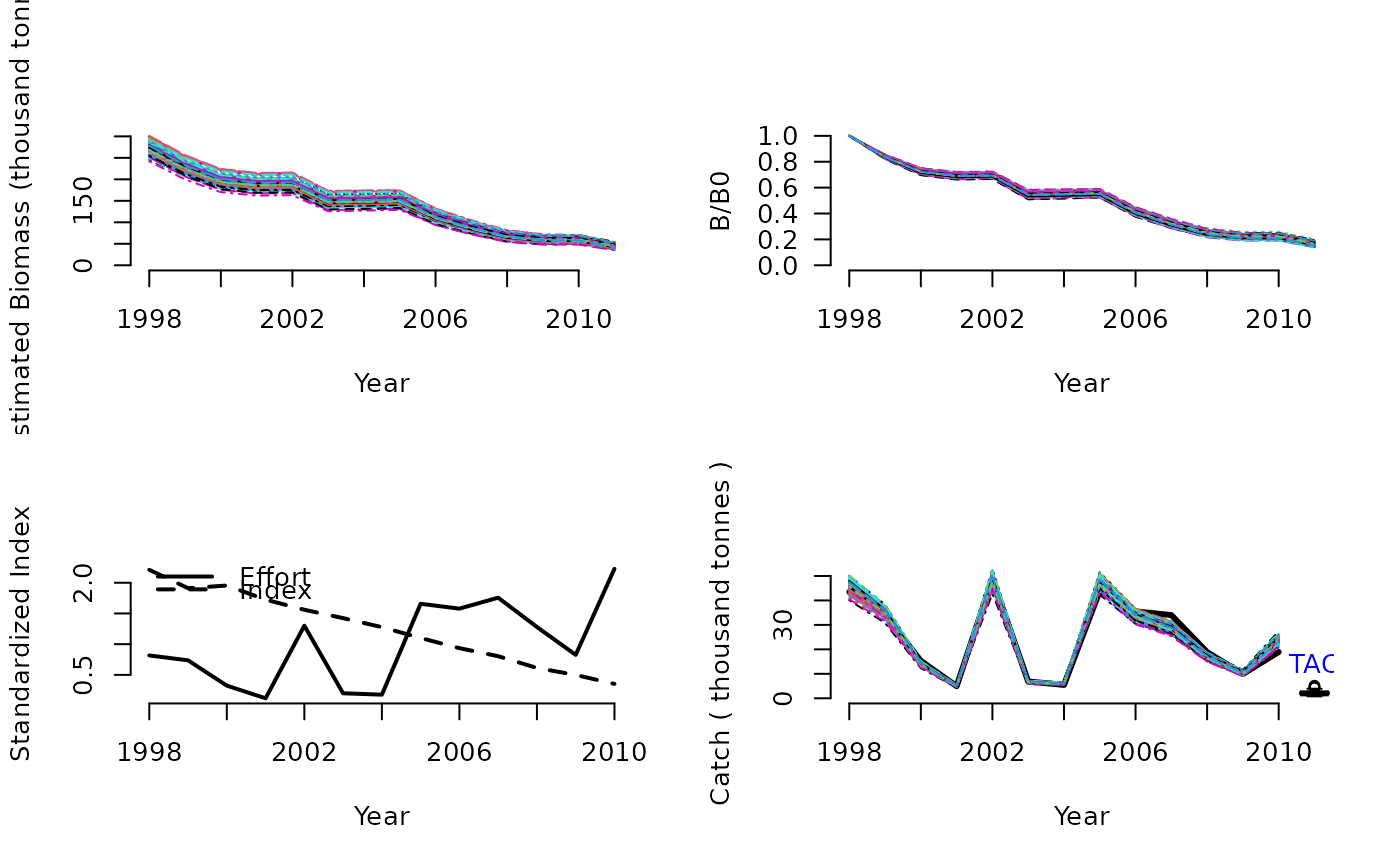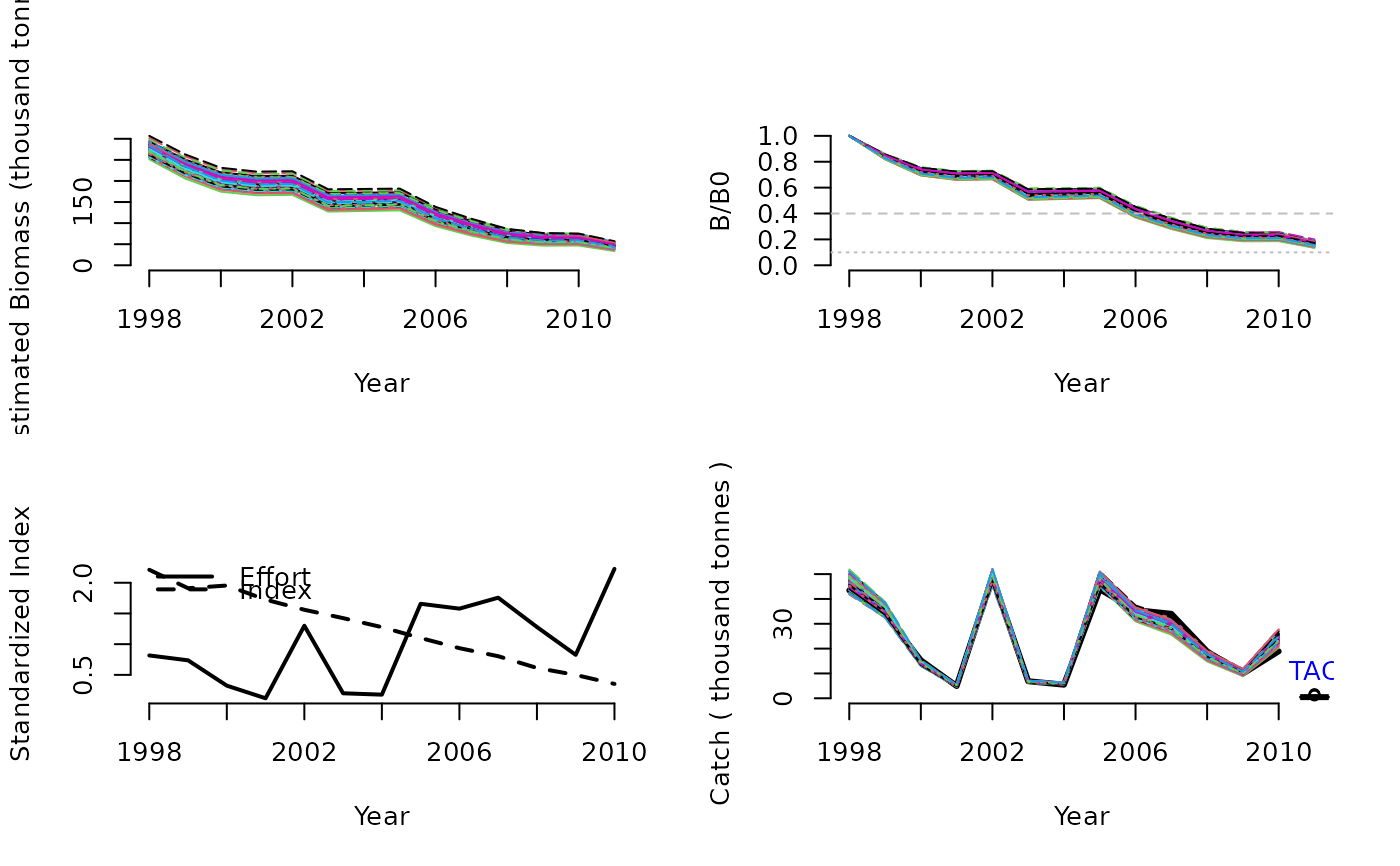A simple delay-difference assessment with UMSY and MSY as leading parameters that estimates the TAC using a time-series of catches and a relative

DD(x, Data, reps = 100, plot = FALSE)

DD4010(x, Data, reps = 100, plot = FALSE)

## Arguments

x

A position in the data object

Data

A data object

reps

The number of stochastic samples of the MP recommendation(s)

plot

Logical. Show the plot?

## Value

An object of class Rec-class with the TAC slot populated with a numeric vector of length reps

## Details

This DD model is observation error only and has does not estimate process error (recruitment deviations). Assumption is that knife-edge selectivity occurs at the age of 50% maturity. Similar to many other assessment models it depends on a whole host of dubious assumptions such as temporally stationary productivity and proportionality between the abundance index and real abundance. Unsurprisingly the extent to which these assumptions are violated tends to be the biggest driver of performance for this method.

The method is conditioned on effort and estimates catch. The effort is calculated as the ratio of catch and index. Thus, to get a complete effort time series, a full time series of catch and index is also needed. Missing values are linearly interpolated.

A detailed description of the delay-difference model can be found in Chapter 9 of Hilborn and Walters (1992).

## Functions

• DD: Base version where the TAC = UMSY * Current Biomass.

• DD4010: A 40-10 rule is imposed over the TAC recommendation.

## Required Data

See Data-class for information on the Data object

DD: Cat, Ind, L50, MaxAge, Mort, vbK, vbLinf, vbt0, wla, wlb

DD4010: Cat, Ind, L50, MaxAge, Mort, vbK, vbLinf, vbt0, wla, wlb

## Rendered Equations

See Online Documentation for correctly rendered equations

Other Delay-Difference MPs: DDe()

T. Carruthers

## Examples

DD(1, Data=MSEtool::Atlantic_mackerel, plot=TRUE)#> TAC (median)
#>     2.077821
DD4010(1, Data=MSEtool::Atlantic_mackerel, plot=TRUE)#> TAC (median)
#>    0.4735525